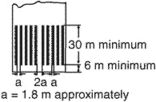# threshold

(redirected from sensing threshold)
Also found in: Dictionary, Thesaurus, Medical, Financial.

## threshold

1. Psychol the strength at which a stimulus is just perceived
2.
a. the minimum intensity or value of a signal, etc., that will produce a response or specified effect
b. (as modifier): a threshold current

## Threshold

A strip fastened to the floor beneath a door, to cover the joint where two types of floor materials meet or to provide weather protection.

## What does it mean when you dream about a threshold?

A threshold is a symbol for passing from one state or condition to the next, indicating a transition in some aspect of the dreamer’s life.

## threshold

[′thresh‚hōld]
(building construction)
A piece of stone, wood, or metal that lies under an outside door.
(electronics)
In a modulation system, the smallest value of carrier-to-noise ratio at the input to the demodulator for all values above which a small percentage change in the input carrier-to-noise ratio produces a substantially equal or smaller percentage change in the output signal-to-noise ratio.
(engineering)
The least value of a current, voltage, or other quantity that produces the minimum detectable response in an instrument or system.
(geology)
(mathematics)
A logic operator such that, if P, Q, R, S, … are statements, then the threshold will be true if at least N statements are true, false otherwise.
(physics)
The minimum level of some input quantity needed for some process to take place, such as a threshold energy for a reaction, or the minimum level of pumping at which a laser can go into self-excited oscillation.
(physiology)
The minimum level of a stimulus that will evoke a response in an irritable tissue.

## threshold

1. A strip fastened to the floor beneath a door, usually required to cover the joint where two types of floor material meet; may provide weather protection at exterior doors. Also See doorsill.
2. In illumination engineering, the value of physical stimulus which permits an object to be seen a specified percentage of the time with specified accuracy.

## thresholdThe beginning of that portion of the runway usable for landing (ICAO). On instrument runways, it is indicated by long stripes followed by the runway designation marking. The number of stripes is dependent on the width of the runway.

## threshold

(1) The point at which a signal (voltage, current, etc.) is perceived as valid.

(2) (Threshold) The code name for Windows 10.
References in periodicals archive ?
(4) [p.sub.busy]-carrier sensing range is the carrier sensing range defined in the static channel multiplied by some factor [a.sub.2.sup.1/[alpha]], where [a.sub.2] [??] [a.sub.2]([p.sub.busy]) is independent of transmission power, carrier sensing threshold, or the path-loss exponent [alpha].
Here, topologically relevant parameters are link distance, path-loss exponent, shadowing factor (the shadowing factor is like stretching or shrinking the link distance, so we can attribute it to the case of link distance), carrier sensing threshold, and transmission power, which are related to interference range or carrier sensing range as well as the accompanying probabilities of [p.sub.fail] or [p.sub.busy].
Caption: Figure 5: Average sensing accuracy as a function of carrier sensing threshold for 802.11b/g 11Mbps ([alpha] = 3, [d.sub.TR] = 10 m, [theta] = 0: (1/12)[pi]: (11/12)[pi], and packet size is 256 bytes).
Sheng and Vastola incorporated the outage probability of carrier sensing into Bianchi's model for the Rayleigh channel , where sensing error was determined by fading, instead of a predefined constant [12-14] or due to improperly set carrier sensing thresholds  assumed in previous works.
(20) attempts to bifurcate the two distributions by taking their point of intersection as the sensing threshold [MATHEMATICAL EXPRESSION NOT REPRODUCIBLE IN ASCII].
In order to achieve simultaneous reduction in the error in detecting joint distributions p(D, [h.sub.i]), we devise the sensing threshold [[lambda].sub.Opt] by equating the error induced in both cases.
The results for our proposed approach of selecting [[lambda].sub.0pt] as the sensing threshold have been displayed in Figure 7.
Through the use of the proposed sensing threshold, spectrum sensing error can be minimized while satisfying spectrum sensing requirement.

Site: Follow: Share:
Open / Close